# 5.4 Discussion and Exercises

Hash tables and hash codes represent an enormous and active field of research that is just touched upon in this chapter. The online Bibliography on Hashing  contains nearly 2000 entries.

A variety of different hash table implementations exist. The one described in Section 5.1 is known as hashing with chaining (each array entry contains a chain (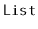) of elements). Hashing with chaining dates back to an internal IBM memorandum authored by H. P. Luhn and dated January 1953. This memorandum also seems to be one of the earliest references to linked lists.

An alternative to hashing with chaining is that used by open addressing schemes, where all data is stored directly in an array. These schemes include thestructure of Section 5.2. This idea was also proposed, independently, by a group at IBM in the 1950s. Open addressing schemes must deal with the problem of collision resolution: the case where two values hash to the same array location. Different strategies exist for collision resolution; these provide different performance guarantees and often require more sophisticated hash functions than the ones described here.

Yet another category of hash table implementations are the so-called perfect hashing methods. These are methods in which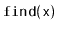operations take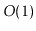time in the worst-case. For static data sets, this can be accomplished by finding perfect hash functions for the data; these are functions that map each piece of data to a unique array location. For data that changes over time, perfect hashing methods include FKS two-level hash tables [31,24] and cuckoo hashing .

The hash functions presented in this chapter are probably among the most practical methods currently known that can be proven to work well for any set of data. Other provably good methods date back to the pioneering work of Carter and Wegman who introduced the notion of universal hashing and described several hash functions for different scenarios . Tabulation hashing, described in Section 5.2.3, is due to Carter and Wegman , but its analysis, when applied to linear probing (and several other hash table schemes) is due to P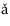tracu and Thorup .

The idea of multiplicative hashing is very old and seems to be part of the hashing folklore [48, Section 6.4]. However, the idea of choosing the multiplier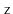to be a random odd number, and the analysis in Section 5.1.1 is due to Dietzfelbinger et al. . This version of multiplicative hashing is one of the simplest, but its collision probability of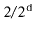is a factor of two larger than what one could expect with a random function from. The multiply-add hashing method uses the functionwhereandare each randomly chosen from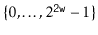. Multiply-add hashing has a collision probability of only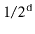, but requires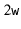-bit precision arithmetic.

There are a number of methods of obtaining hash codes from fixed-length sequences of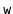-bit integers. One particularly fast method  is the functionwhere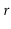is even and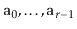are randomly chosen from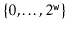. This yields a-bit hash code that has collision probability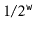. This can be reduced to a-bit hash code using multiplicative (or multiply-add) hashing. This method is fast because it requires only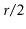-bit multiplications whereas the method described in Section 5.3.2 requiresmultiplications. (Theoperations occur implicitly by usingand-bit arithmetic for the additions and multiplications, respectively.)

The method from Section 5.3.3 of using polynomials over prime fields to hash variable-length arrays and strings is due to Dietzfelbinger et al. . Due to its use of theoperator which relies on a costly machine instruction, it is, unfortunately, not very fast. Some variants of this method choose the prime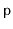to be one of the form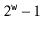, in which case the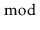operator can be replaced with addition () and bitwise-and (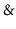) operations [47, Section 3.6]. Another option is to apply one of the fast methods for fixed-length strings to blocks of length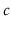for some constant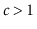and then apply the prime field method to the resulting sequence of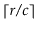hash codes.

Exercise 5..1   A certain university assigns each of its students student numbers the first time they register for any course. These numbers are sequential integers that started at 0 many years ago and are now in the millions. Suppose we have a class of one hundred first year students and we want to assign them hash codes based on their student numbers. Does it make more sense to use the first two digits or the last two digits of their student number? Justify your answer.

Exercise 5..2   Consider the hashing scheme in Section 5.1.1, and suppose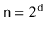and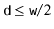.
1. Show that, for any choice of the muliplier,, there exists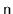values that all have the same hash code. (Hint: This is easy, and doesn't require any number theory.)
2. Given the multiplier,, describevalues that all have the same hash code. (Hint: This is harder, and requires some basic number theory.)

Exercise 5..3   Prove that the boundin Lemma 5.1 is the best possible bound by showing that, if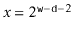and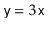, then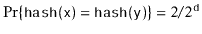. (Hint look at the binary representations of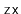and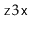and use the fact that.)

Exercise 5..4   Reprove Lemma 5.4 using the full version of Stirling's Approximation given in Section 1.3.2.

Exercise 5..5   Consider the following simplified version of the code for adding an element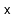to a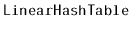, which simply storesin the first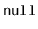array entry it finds. Explain why this could be very slow by giving an example of a sequence of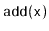,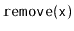, andoperations that would take on the order of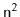time to execute.
  bool addSlow(T x) {
if (2*(q+1) > t.length) resize();   // max 50% occupancy
int i = hash(x);
while (t[i] != null) {
if (t[i] != del && x.equals(t[i])) return false;
i = (i == t.length-1) ? 0 : i + 1; // increment i
}
t[i] = x;
n++; q++;
return true;
}


Exercise 5..6   Early versions of the Java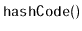method for the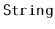class worked by not using all of the characters found in long strings. For example, for a sixteen character string, the hash code was computed using only the eight even-indexed characters. Explain why this was a very bad idea by giving an example of large set of strings that all have the same hash code.

Exercise 5..7   Suppose you have an object made up of two-bit integers,and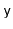. Show why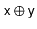does not make a good hash code for your object. Give an example of a large set of objects that would all have hash code 0.

Exercise 5..8   Suppose you have an object made up of two-bit integers,and. Show why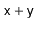does not make a good hash code for your object. Give an example of a large set of objects that would all have the same hash code.

Exercise 5..9   Suppose you have an object made up of two-bit integers,and. Suppose that the hash code for your object is defined by some deterministic functionthat produces a single-bit integer. Prove that there exists a large set of objects that have the same hash code.

Exercise 5..10   Let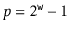for some positive integer. Explain why, for a positive integer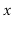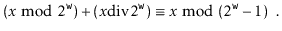(This gives an algorithm for computingby repeatedly setting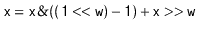until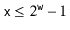.)

Exercise 5..11   Find some commonly used hash table implementation such as the (The C++ STL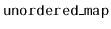or the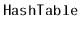orimplementations in this book, and design a program that stores integers in this data structure so that there are integers,, such thattakes linear time. That is, find a set ofintegers for which there are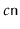elements that hash to the same table location.

Depending on how good the implementation is, you may be able to do this just by inspecting the code for the implementation, or you may have to write some code that does trial insertions and searches, timing how long it takes to add and find particular values. (This can be, and has been, used to launch denial of service attacks on web servers .)

opendatastructures.org